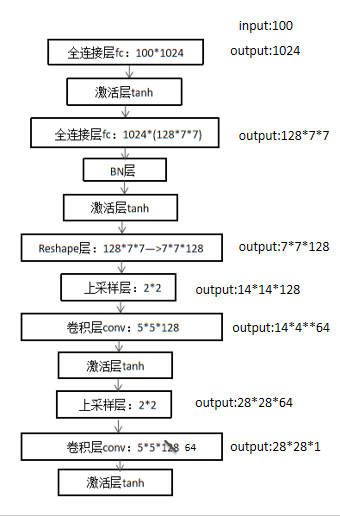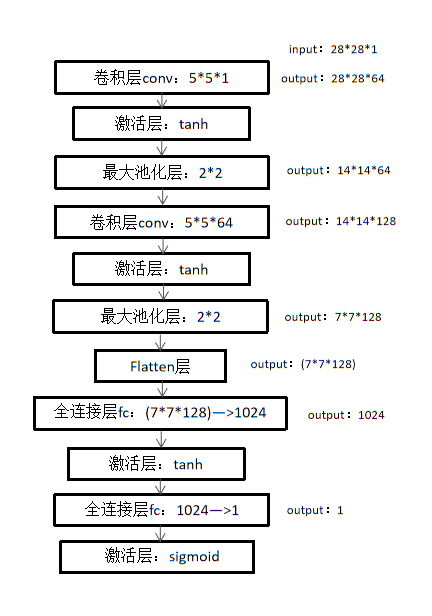1. 导入部分：

from keras.models import Sequential
from keras.layers import Dense
from keras.layers import Reshape
from keras.layers.core import Activation
from keras.layers.normalization import BatchNormalization
from keras.layers.convolutional import UpSampling2D
from keras.layers.convolutional import Conv2D, MaxPooling2D
from keras.layers.core import Flatten
from keras.optimizers import SGD
from keras.datasets import mnist
import numpy as np
from PIL import Image
import argparse
import math

PIL：Python Imaging Library，python中的图像处理库，看介绍就是有很强的图像处理能力，隐约中记得安装过；

argparse：还不太懂，貌似是能在命令行中提供更加用户友好的参数选择接口。

2. 生成器网络结构：

def generator_model():
#下面搭建生成器的架构，首先导入序贯模型（sequential），即多个网络层的线性堆叠
model = Sequential()
#添加一个全连接层，输入为100维向量，输出为1024维
#添加一个激活函数tanh
#添加一个全连接层，输出为128×7×7维度
#添加一个批量归一化层，该层在每个batch上将前一层的激活值重新规范化，即使得其输出数据的均值接近0，其标准差接近1
#Reshape层用来将输入shape转换为特定的shape，将含有128*7*7个元素的向量转化为7×7×128张量
#2维上采样层，即将数据的行和列分别重复2次
#卷积核设为1即输出图像的维度
return modelMNIST数据集的图片大小就是28*28*1，说明以上output的推导没有问题，生成器的输出图片大小应该和MNIST中真实的图片大小相同。

Questions：

1）为什么激活函数选择tanh；

2）卷积层的作用：还是提取特征吗，理论上怎么理解啊，感觉和一般的图像分类的网络比是反过来了，有点晕。

3. 判别器网络结构：

def discriminator_model():
#下面搭建判别器架构，同样采用序贯模型
model = Sequential()

#添加2维卷积层，卷积核大小为5×5，激活函数为tanh，输入shape在‘channels_first’模式下为（samples,channels，rows，cols）
#在‘channels_last’模式下为（samples,rows,cols,channels），输出为64维
Conv2D(64, (5, 5),
input_shape=(28, 28, 1))
)
#为空域信号施加最大值池化，pool_size取（2，2）代表使图片在两个维度上均变为原长的一半
#Flatten层把多维输入一维化，常用在从卷积层到全连接层的过渡
#一个结点进行二值分类，并采用sigmoid函数的输出作为概念
return model4. 对抗网络结构：

def generator_containing_discriminator(g, d):
#将前面定义的生成器架构和判别器架构组拼接成一个大的神经网络，用于判别生成的图片
model = Sequential()
#先添加生成器架构，再令d不可训练，即固定d
#因此在给定d的情况下训练生成器，即通过将生成的结果投入到判别器进行辨别而优化生成器
d.trainable = False
return model

5. 生成图片拼接：

def combine_images(generated_images):
#生成图片拼接
num = generated_images.shape
width = int(math.sqrt(num))
height = int(math.ceil(float(num)/width))
shape = generated_images.shape[1:3]
image = np.zeros((height*shape, width*shape),
dtype=generated_images.dtype)
for index, img in enumerate(generated_images):
i = int(index/width)
j = index % width
image[i*shape:(i+1)*shape, j*shape:(j+1)*shape] = \
img[:, :, 0]
return image

6. 对抗网络训练：

def train(BATCH_SIZE):

# 国内好像不能直接导入数据集，我们试了几次都不行，后来将数据集下载到本地'~/.keras/datasets/'，也就是当前目录（我的是用户文件夹下）下的.keras文件夹中。
#下载的地址为：https://s3.amazonaws.com/img-datasets/mnist.npz
(X_train, y_train), (X_test, y_test) = mnist.load_data()
#iamge_data_format选择"channels_last"或"channels_first"，该选项指定了Keras将要使用的维度顺序。
#"channels_first"假定2D数据的维度顺序为(channels, rows, cols)，3D数据的维度顺序为(channels, conv_dim1, conv_dim2, conv_dim3)

#转换字段类型，并将数据导入变量中
X_train = (X_train.astype(np.float32) - 127.5)/127.5
X_train = X_train[:, :, :, None]
X_test = X_test[:, :, :, None]
# X_train = X_train.reshape((X_train.shape, 1) + X_train.shape[1:])

#将定义好的模型架构赋值给特定的变量
d = discriminator_model()
g = generator_model()
d_on_g = generator_containing_discriminator(g, d)

#定义生成器模型判别器模型更新所使用的优化算法及超参数
d_optim = SGD(lr=0.001, momentum=0.9, nesterov=True)
g_optim = SGD(lr=0.001, momentum=0.9, nesterov=True)

#编译三个神经网络并设置损失函数和优化算法，其中损失函数都是用的是二元分类交叉熵函数。编译是用来配置模型学习过程的
g.compile(loss='binary_crossentropy', optimizer="SGD")
d_on_g.compile(loss='binary_crossentropy', optimizer=g_optim)

#前一个架构在固定判别器的情况下训练了生成器，所以在训练判别器之前先要设定其为可训练。
d.trainable = True
d.compile(loss='binary_crossentropy', optimizer=d_optim)

#下面在满足epoch条件下进行训练
for epoch in range(30):
print("Epoch is", epoch)

#计算一个epoch所需要的迭代数量，即训练样本数除批量大小数的值取整；其中shape就是读取矩阵第一维度的长度
print("Number of batches", int(X_train.shape/BATCH_SIZE))

#在一个epoch内进行迭代训练
for index in range(int(X_train.shape/BATCH_SIZE)):

#随机生成的噪声服从均匀分布，且采样下界为-1、采样上界为1，输出BATCH_SIZE×100个样本；即抽取一个批量的随机样本
noise = np.random.uniform(-1, 1, size=(BATCH_SIZE, 100))

#抽取一个批量的真实图片
image_batch = X_train[index*BATCH_SIZE:(index+1)*BATCH_SIZE]

#生成的图片使用生成器对随机噪声进行推断；verbose为日志显示，0为不在标准输出流输出日志信息，1为输出进度条记录
generated_images = g.predict(noise, verbose=0)

#每经过100次迭代输出一张生成的图片
if index % 100 == 0:
image = combine_images(generated_images)
image = image*127.5+127.5
Image.fromarray(image.astype(np.uint8)).save(
"./GAN/"+str(epoch)+"_"+str(index)+".png")

#将真实的图片和生成的图片以多维数组的形式拼接在一起，真实图片在上，生成图片在下
X = np.concatenate((image_batch, generated_images))

#生成图片真假标签，即一个包含两倍批量大小的列表；前一个批量大小都是1，代表真实图片，后一个批量大小都是0，代表伪造图片
y =  * BATCH_SIZE +  * BATCH_SIZE

#判别器的损失；在一个batch的数据上进行一次参数更新
d_loss = d.train_on_batch(X, y)
print("batch %d d_loss : %f" % (index, d_loss))

#随机生成的噪声服从均匀分布
noise = np.random.uniform(-1, 1, (BATCH_SIZE, 100))

#固定判别器
d.trainable = False

#计算生成器损失；在一个batch的数据上进行一次参数更新
g_loss = d_on_g.train_on_batch(noise,  * BATCH_SIZE)

#令判别器可训练
d.trainable = True
print("batch %d g_loss : %f" % (index, g_loss))

#每100次迭代保存一次生成器和判别器的权重
if index % 100 == 9:
g.save_weights('generator', True)
d.save_weights('discriminator', True)

X_train = (X_train.astype(np.float32) - 127.5)/127.5
X_train = X_train[:, :, :, None]
X_test = X_test[:, :, :, None]
# X_train = X_train.reshape((X_train.shape, 1) + X_train.shape[1:])


g.compile(loss='binary_crossentropy', optimizer="SGD")
d_on_g.compile(loss='binary_crossentropy', optimizer=g_optim)
d.trainable = True
d.compile(loss='binary_crossentropy', optimizer=d_optim)

print("Number of batches", int(X_train.shape/BATCH_SIZE))

if index % 100 == 0:
image = combine_images(generated_images)
image = image*127.5+127.5
Image.fromarray(image.astype(np.uint8)).save( "./GAN/"+str(epoch)+"_"+str(index)+".png")

X = np.concatenate((image_batch, generated_images))
y =  * BATCH_SIZE +  * BATCH_SIZE
d_loss = d.train_on_batch(X, y)

d.trainable = False
g_loss = d_on_g.train_on_batch(noise,  * BATCH_SIZE)
d.trainable = True
print("batch %d g_loss : %f" % (index, g_loss))

每迭代100次保存一下权重，保存权重的次数(index % 10 == 9)刚好是用来产生上面输出图片(index % 10 == 0)的前一次，就是产生那些图片的权重：

if index % 100 == 9:
g.save_weights('generator', True)
d.save_weights('discriminator', True)

7. 生成图片：

def generate(BATCH_SIZE, nice= False ):
#训练完模型后，可以运行该函数生成图片
g = generator_model()
g.compile(loss='binary_crossentropy', optimizer="SGD")
if nice:
d = discriminator_model()
d.compile(loss='binary_crossentropy', optimizer="SGD")
noise = np.random.uniform(-1, 1, (BATCH_SIZE*20, 100))
generated_images = g.predict(noise, verbose=1)
d_pret = d.predict(generated_images, verbose=1)
index = np.arange(0, BATCH_SIZE*20)
index.resize((BATCH_SIZE*20, 1))
pre_with_index = list(np.append(d_pret, index, axis=1))
pre_with_index.sort(key=lambda x: x, reverse=True)
nice_images = np.zeros((BATCH_SIZE,) + generated_images.shape[1:3], dtype=np.float32)
nice_images = nice_images[:, :, :, None]
for i in range(BATCH_SIZE):
idx = int(pre_with_index[i])
nice_images[i, :, :, 0] = generated_images[idx, :, :, 0]
image = combine_images(nice_images)
else:
noise = np.random.uniform(-1, 1, (BATCH_SIZE, 100))
generated_images = g.predict(noise, verbose=0)
image = combine_images(generated_images)
image = image*127.5+127.5
Image.fromarray(image.astype(np.uint8)).save(
"./GAN/generated_image.png")